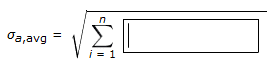# Uncertainty of Average Acceleration

pry_or

## Homework Statement

You have determined the acceleration $a_{i}$ of an object in free fall for i = 1 to n different time intervals. What is the algebraic expression for the uncertainty $σ_{a,avg}$ in $a_{avg}$? (Use any variable or symbol stated above as necessary.)

The answer box is formatted as such: $σ_{a,avg}$ = $\sqrt{\sum^{i=1}_{n}}$

and I have to fill in the space after the summation under the square root.

No variables or symbols were specifically given for the problem outside of the ones in the question, but I had written the formula for average acceleration previously (in the relevant equations section) that may have variables I can use.

## Homework Equations

$a_{avg}$ = $\frac{v_{23} - v_{12}}{t_{23} - t_{12}}$

## The Attempt at a Solution

I honestly have no clue where to begin. I don't really have much knowledge of uncertainty calculations and so I am pretty lost here. I tried one method first that I realized is incorrect.

Any help would be extremely appreciated.

Muphrid
Do you know the definition of the standard deviation $\sigma$ or the variance $\sigma^2$? It seems unusual to be asked such a question without at least some reference to the definition in the text.

pry_or
Yeah, I know the definitions. They are given to me. I can usually apply the formulas, but I am not sure how to do it for this question.

After some thinking, I believe I could write the formula a = Δv/Δt, since I can calculate $σ_{Δv}$ and $σ_{Δt}$ using basic addition. So $σ_{Δv} = σ_{v_{12}} + σ_{v_{23}}$ and $σ_{Δt} = σ_{t_{12}} + σ_{t_{23}}$. So I can then apply it to the multiplication formula to find the uncertainty for a. Using the formula given in the text, I would think the uncertainty for the basic formula I gave in my original post would be

$σ_{a_{avg}} = a_{avg}\sqrt{(\frac{σ_{Δv}}{Δv})^{2} + (\frac{-σ_{Δt}}{Δt})^{2}}$

I don't know whether this is correct or not. And if it is, I am stuck trying to figure out how to use the summation that is given in the answer area.

For ease of reference, here is the answer area:Muphrid
Yeah, you're kind of going off into space here. This problem isn't about dealing with uncertainties from other variables, just from the data set that you have.

Something like this formula should look familiar:

$$\sigma_x^2 = \sum_i (x_i - \bar x)^2/N$$

Last edited:
pry_or
It's been about 2.5 years since I did real standard deviation or variance calculations. The two formulas given in the text are both about uncertainties from other variables. One is for addition of uncertainties and one is for products of uncertainties. And in previous assignments, all the calculations we did dealt with these formulas. This is what is throwing me off because the book doesn't include anything regarding the answer formula. And as such, I do not fully understand your formula. I believe it looks familiar from my previous math experience though.

So I am guessing $x_{i}$ would be $a_{i}$ and $/bar{x}$ would be $a_{avg}$?

Muphrid
Yes. You might also see $\sigma^2_x = \langle x^2 \rangle - \langle x \rangle^2$. Also note that I neglected a factor of $N$ which is necessary to to get it right.

pry_or
$$\sigma_x^2 = \sum_i (x_i - \bar x)^2/N$$

Alright, this is the answer after all. Even though there is not a single reference to this in the book! Thanks very much for your help!